Search by Topic

Resources tagged with Topology similar to Torus Patterns:

Filter by: Content type:
Age range:
Challenge level:

There are 14 results

Broad Topics > Decision Mathematics and Combinatorics > Topology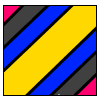Torus Patterns

Age 16 to 18 Challenge Level:

How many different colours would be needed to colour these different patterns on a torus?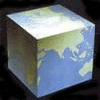Earth Shapes

Age 16 to 18 Challenge Level:

What if the Earth's shape was a cube or a cone or a pyramid or a saddle ... See some curious worlds here.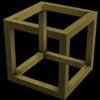Impossible Polyhedra

Age 16 to 18

Is it possible to make an irregular polyhedron using only polygons of, say, six, seven and eight sides? The answer (rather surprisingly) is 'no', but how do we prove a statement like this?A Curious Collection of Bridges

Age 11 to 18

Read about the problem that tickled Euler's curiosity and led to a new branch of mathematics!Tangles

Age 11 to 16

A personal investigation of Conway's Rational Tangles. What were the interesting questions that needed to be asked, and where did they lead?Geometry and Gravity 1

Age 11 to 18

This article (the first of two) contains ideas for investigations. Space-time, the curvature of space and topology are introduced with some fascinating problems to explore.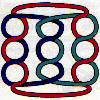Age 14 to 18

Some puzzles requiring no knowledge of knot theory, just a careful inspection of the patterns. A glimpse of the classification of knots, prime knots, crossing numbers and knot arithmetic.Where Do We Get Our Feet Wet?

Age 16 to 18

Professor Korner has generously supported school mathematics for more than 30 years and has been a good friend to NRICH since it started.Symmetric Tangles

Age 14 to 16

The tangles created by the twists and turns of the Conway rope trick are surprisingly symmetrical. Here's why!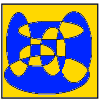Painting by Numbers

Age 16 to 18 Challenge Level:

How many different colours of paint would be needed to paint these pictures by numbers?Geometry and Gravity 2

Age 11 to 18

This is the second of two articles and discusses problems relating to the curvature of space, shortest distances on surfaces, triangulations of surfaces and representation by graphs.Euler's Formula and Topology

Age 16 to 18

Here is a proof of Euler's formula in the plane and on a sphere together with projects to explore cases of the formula for a polygon with holes, for the torus and other solids with holes and the. . . .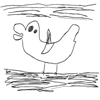The Development of Spatial and Geometric Thinking: 5 to 18

Age 5 to 16

This is the first article in a series which aim to provide some insight into the way spatial thinking develops in children, and draw on a range of reported research. The focus of this article is the. . . .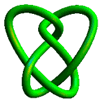The Invertible Trefoil

Age 14 to 16 Challenge Level:

When is a knot invertible ?